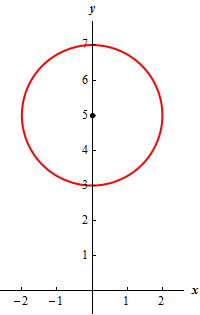Paul's Online Notes
Home / Algebra / Graphing and Functions / Circles
Show Mobile Notice Show All Notes Hide All Notes
Mobile Notice
You appear to be on a device with a "narrow" screen width (i.e. you are probably on a mobile phone). Due to the nature of the mathematics on this site it is best views in landscape mode. If your device is not in landscape mode many of the equations will run off the side of your device (should be able to scroll to see them) and some of the menu items will be cut off due to the narrow screen width.

### Section 3.3 : Circles

4. Determine the radius and center of the following circle and sketch the graph of the circle.

${x^2} + {\left( {y - 5} \right)^2} = 4$

Show All Steps Hide All Steps

Start Solution

There really isn’t much to this problem. All we need to do is compare the equation we’ve been given to the standard form of the circle to determine the radius and center of the circle. For reference purposes here is the standard form of the circle.

${\left( {x - h} \right)^2} + {\left( {y - k} \right)^2} = {r^2}$

First, notice that we can write the first term as,

${x^2} = {\left( {x - 0} \right)^2}$

and so we can now see that $$h = 0$$

Next, from the second term we can quickly see that $$k = 5$$.

Finally, we see that $${r^2} = 4$$ and so we must have $$r = 2$$ .

Therefore, the radius of the circle is 2 and the center of the circle is $$\left( {0,5} \right)$$ .

Show Step 2

Here is a sketch of the circle.Remember that we can quickly graph the circle by starting at the center and then going right/left by the radius (2 for this problem) to get the right most/left most points. To get the top most/bottom most point all we need to do is start at the center and go up/down by the radius (again, 2 for this problem).